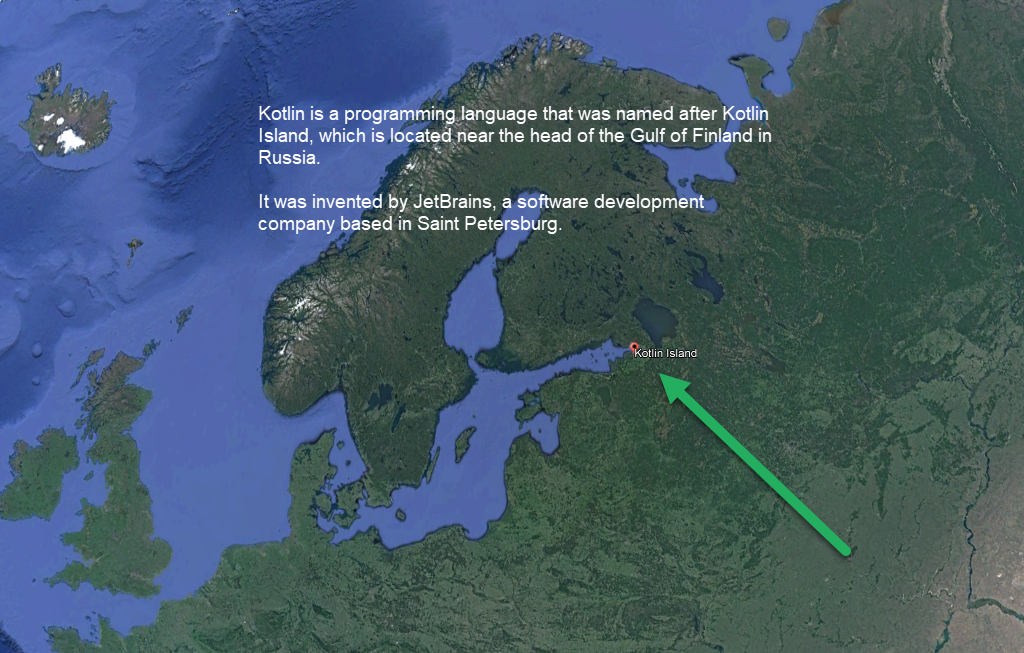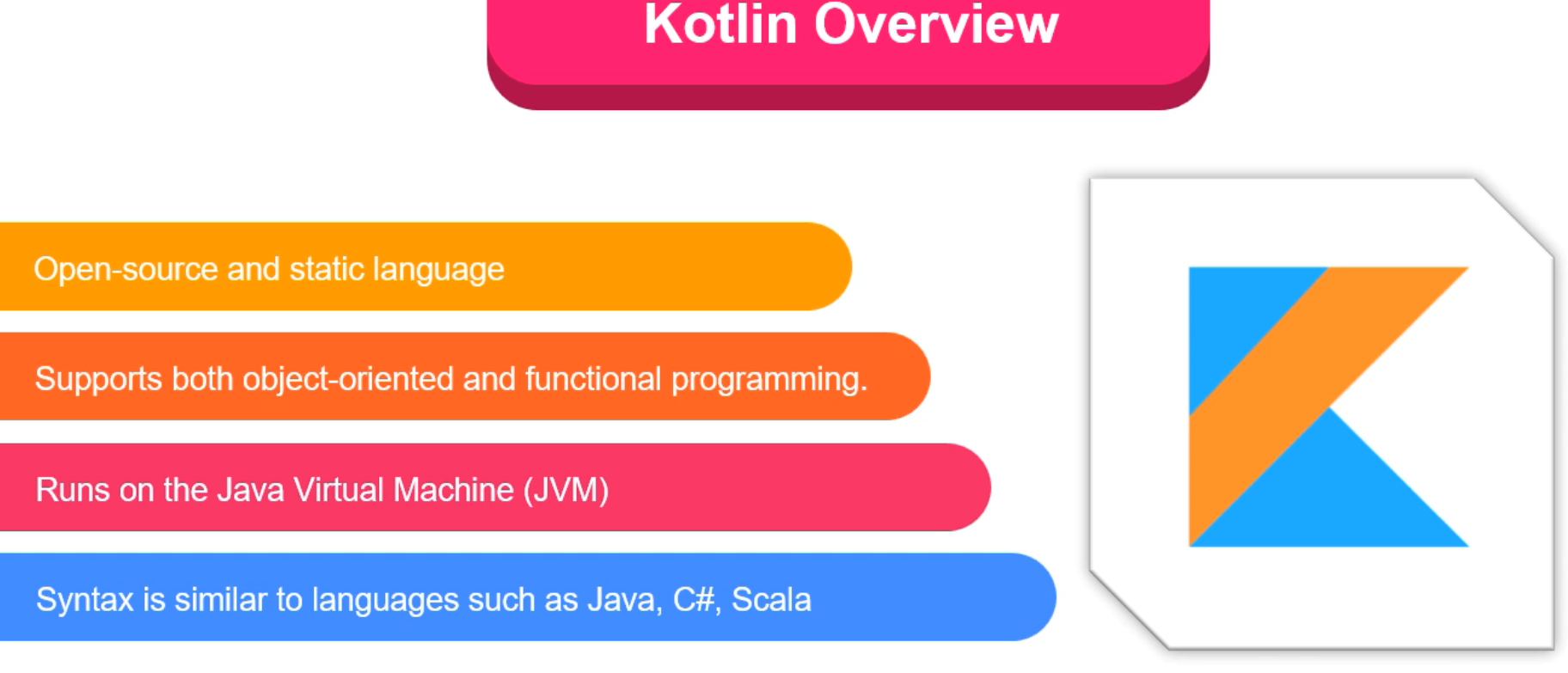KOTLIN LAB BOOK
Share
Explore# Lecture Summary

## Session 01: Tuesday Feb 21

Topics covered:
Familiarizing yourself with Intellij IDEA
Kotlin Syntax
The main() function (Activity #A)
Creating variables with val and var
Output to console with println and \$ symbol
Conditionals
For loop, while loop
Declaring and using a function with and without parameters
Fixed size arrays (arrayOf), dynamically sized arrays (listOf)
Looping through an array (Activity #D)
How to generate random numbers

## Session 02: Wed Feb 22:

Topics Discussed:
Declaring a class and its constructor
What is. constructor, how is it used
What are class properties and how are they used
Providing a default value to a class property
Developing class-oriented programs (Example: )

# Session 01 - Tuesday Feb 21

## Installing IntelliJ

Kotlin is a popular programming language that supports various programming paradigms, including object-oriented and functional programming.

Looping enables developers to iterate over a set of values or execute a block of code repeatedly. Here are the types of loops available in Kotlin:
For loop: A for loop in Kotlin is used to iterate over a range, an array, a collection, or any object that provides an iterator. The syntax of the for loop is similar to that of Java and other programming languages. You can also use a for loop to iterate over a string using the indices property.
While loop: A while loop in Kotlin is used to execute a block of code repeatedly while a specified condition is true. The while loop evaluates the condition before each iteration and stops when the condition becomes false.
Do-While loop: The do-while loop in Kotlin is similar to the while loop, except that it executes the block of code at least once, even if the condition is false. The condition is evaluated after each iteration, and the loop stops when the condition becomes false.

## Activity A

Here's a Kotlin code example that adds two numbers together and prints the result to the console:
```fun main() { val num1 = 5 val num2 = 7 val sum = num1 + num2 println("The sum of \$num1 and \$num2 is \$sum") }```
This program declares two variables num1 and num2, each containing an integer value. It then declares a third variable sum that is the result of adding num1 and num2. Finally, it prints a message to the console using string interpolation to display the values of num1, num2, and sum.

## Activity B: Game Scenario: Guess the Number

In this game, the program chooses a random number between 1 and 10, and the player has to guess the number. If the player's guess is correct, they win the game. If their guess is incorrect, the program tells them whether the actual number is higher or lower than their guess, and they get to guess again. The game ends after the player correctly guesses the number or after they have made 3 incorrect guesses.
This game scenario is simple enough to be implemented using basic Kotlin programming concepts like random number generation, user input, conditional statements, and loops. It can be a good introduction to the language for beginners who are just starting out.

`import kotlin.random.Random````fun main() { val secretNumber = Random.nextInt(1, 11) var numGuesses = 0 var guessedCorrectly = false println("Welcome to Guess the Number! You have 3 attempts to guess the number between 1 and 10.") while (numGuesses < 3 && !guessedCorrectly) { print("Guess #\$numGuesses: ") val guess = readLine()?.toIntOrNull() if (guess == null) { println("Invalid input. Please enter a number between 1 and 10.") } else if (guess == secretNumber) { guessedCorrectly = true println("Congratulations, you guessed the number!") } else if (guess < secretNumber) { println("Your guess is too low. Try again.") } else { println("Your guess is too high. Try again.") } numGuesses++ } if (!guessedCorrectly) { println("Sorry, you did not guess the number. The secret number was \$secretNumber.") } }```

The program starts by generating a random number between 1 and 10 using the Random.nextInt() function from the Kotlin standard library. It then initializes two variables: numGuesses to keep track of the number of guesses the player has made, and guessedCorrectly to indicate whether the player has guessed the correct number.
The program then prints a welcome message and enters a while loop that executes as long as the player has made less than 3 incorrect guesses and has not guessed the correct number. Within the loop, the program prompts the player to make a guess and reads the player's input using the readLine() function. It then checks whether the input is a valid integer between 1 and 10 using the toIntOrNull() function. If the input is invalid, the program prints an error message. Otherwise, it checks whether the guess is correct, too low, or too high, and provides feedback to the player accordingly. It also increments the numGuesses counter.
After the loop has ended, the program checks whether the player has guessed the correct number. If they have, it prints a congratulatory message. If they have not, it prints a message indicating that the game is over and reveals the secret number to the player.
This program is relatively simple and uses basic Kotlin programming concepts like while loops, conditional statements (if-else), string interpolation, and the readLine() and toIntOrNull() functions to handle user input.

## Activity C: ROCKS PAPER SCISSORS

The program starts by defining an array of the three possible choices. It then prompts the player to enter their name using the getPlayerName() function, and initializes the scores for both the player and the computer
`import kotlin.random.Random````fun main() { val choices = arrayOf("Rock", "Paper", "Scissors") val playerName = getPlayerName() var playerScore = 0 var computerScore = 0 println("Hello \$playerName, let's play Rock-Paper-Scissors!") while (playerScore < 3 && computerScore < 3) { val playerChoice = getPlayerChoice() val computerChoice = choices.random() println("\$playerName chose \$playerChoice") println("Computer chose \$computerChoice") val roundWinner = determineRoundWinner(playerChoice, computerChoice) if (roundWinner == playerName) { playerScore++ println("\$playerName wins the round!") } else if (roundWinner == "Computer") { computerScore++ println("Computer wins the round!") } else { println("It's a tie!") } println("Score: \$playerName: \$playerScore, Computer: \$computerScore") } if (playerScore > computerScore) { println("\$playerName wins the game!") } else { println("Computer wins the game!") } }``````fun getPlayerName(): String { print("Please enter your name: ") return readLine() ?: "Player" }``````fun getPlayerChoice(): String { print("Rock, Paper, or Scissors? ") var playerChoice = readLine() while (playerChoice !in arrayOf("Rock", "Paper", "Scissors")) { println("Invalid choice. Please choose Rock, Paper, or Scissors.") print("Rock, Paper, or Scissors? ") playerChoice = readLine() } return playerChoice }``````fun determineRoundWinner(playerChoice: String, computerChoice: String): String { if (playerChoice == "Rock" && computerChoice == "Scissors" || playerChoice == "Scissors" && computerChoice == "Paper" || playerChoice == "Paper" && computerChoice == "Rock") { return "Player" } else if (playerChoice == "Rock" && computerChoice == "Paper" || playerChoice == "Scissors" && computerChoice == "Rock" || playerChoice == "Paper" && computerChoice == "Scissors") { return "Computer" } else { return "Tie" } }```

## Activity D: Here's an example Kotlin program that showcases loops, if statements, function calls, arrays, and lists:

`fun main() {`` val myArray = arrayOf(1, 2, 3, 4, 5)`` val myList = listOf("apple", "banana", "cherry")`
` // Loop through an array and print each element`` for (element in myArray) {`` println("Array element: \$element")`` }`
` // Loop through a list and print each element`` for (element in myList) {`` println("List element: \$element")`` }`
` // Use an if statement to check if an array element is even`` for (element in myArray) {`` if (element % 2 == 0) {`` println("\$element is even")`` }`` }`
` // Call a function to print the sum of an array`` val sum = getArraySum(myArray)`` println("Sum of the array: \$sum")``}`
`// Define a function to calculate the sum of an array``fun getArraySum(arr: Array<Int>): Int {`` var sum = 0`` for (element in arr) {`` sum += element`` }`` return sum``}`
In this program, we define an array myArray and a list myList, and then use loops to iterate through each of them and print their elements. We also use an if statement to check if each element of myArray is even. Finally, we call a function getArraySum to calculate the sum of myArray, and print the result.

## More examples of Kotlin functions:

Here are additional code examples of functions can be used

### Activity E: A simple function that takes in two integer parameters and returns their sum:

`fun sum(a: Int, b: Int): Int {`` return a + b``}`
`// Calling the function``val result = sum(2, 3)``println("The result is \$result")`
Output:

`The result is 5`

### Activity F: A function that takes in a string and a default value as parameters, and returns the string if it is not null or empty, otherwise returns the default value:

`fun getStringOrDefault(x: String?, y: String): String {``// if x == null, then "return y"``// if x != null, then "return x"`` return x ?: y``}`
`// Calling the function``val str1 = getStringOrDefault("Hello", "ABC")``val str2 = getStringOrDefault(null, "ABC")``val str3 = getStringOrDefault("", "Default")`
`println(str1) // Output: Hello``println(str2) // Output: ABC``println(str3) // Output: Default`

### Activity G: A function that calculates the factorial of a given number using recursion:

`fun factorial(n: Int): Int {`` if (n == 0 || n == 1) {`` return 1`` }`` return n * factorial(n - 1)``}`
`// Calling the function``val result = factorial(5)``println("The factorial of 5 is \$result")`
Output:

`The factorial of 5 is 120`
In each example, we declare the function using the fun keyword followed by the function name and the function's parameters in parentheses. The function's return type is specified after the parameter list with a colon followed by the type. The function body is enclosed in curly braces. To call the function, we simply use the function name followed by the arguments in parentheses.

## 5 examples of random number generation in Kotlin:

Generate a random integer between 1 and 100:

```fun main() { ​for ( i in (1 .. 100)) { val randomINT = (1..100).random() println(randomINT); } } ```
Generate a random double between 0 and 1:

`val randomDouble = Math.random()`
Generate a random integer within a specific range:

`val range = 10..20``val randomIntInRange = range.random()`
```fun main() { for ( i in (1 .. 100)) { val range = 10..20 val randomIntInRange = range.random() println(randomIntInRange) } } ```
```fun main() { for ( i in (1 .. 100)) { val range = 10..20 val randomIntInRange = range.random() * 5 println(randomIntInRange) } }```
```fun main() { fun printRandomIntegersInStepsOfFive() { for (i in 1..100) { val range = 10..20 val randomIntInRange = range.random() * 5 println(randomIntInRange) } } printRandomIntegersInStepsOfFive() }```
Generate a random boolean:

`val randomBoolean = listOf(true, false).random()`
Generate a random element from an array:

`val items = arrayOf("apple", "banana", "orange")``val randomItem = items.random()`
Note: To generate a random number, you can use the random() function available on different types, like IntRange, Double, List, or Array

## Practice lab Test Question:

Solve the following requirement in KOTLIN:
Imagine Joe - invites you to a little gambling game.
One side of coin : number 5
Other side : number 10
Joe flips : wins double the number showing
You flip: wins double the number showing
game proceeds (3..10) times
keep track of who owns how much to who
printout number net amount owning